• +91 9971497814
• info@interviewmaterial.com

# RD Chapter 1- Number Systems Ex-1.4 Interview Questions Answers

### Related Subjects

Question 1 : Define an irrational number.

Answer 1 : A number which cannot be expressed in the form of pq where p and q are integers and q ≠ 0 is called an irrational number.

Question 2 : Explain, how irrational numbers differ from rational numbers?

Answer 2 : A rational number can be expressed in either terminating decimal or non-terminating recurring decimals but an irrational number is expressed in non-terminating non-recurring decimals.

Question 3 : Examine, whether the following numbers are rational or irrational: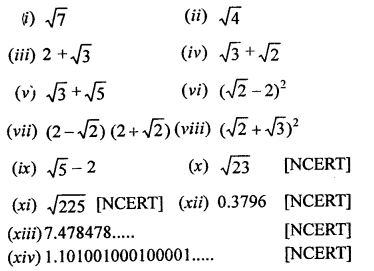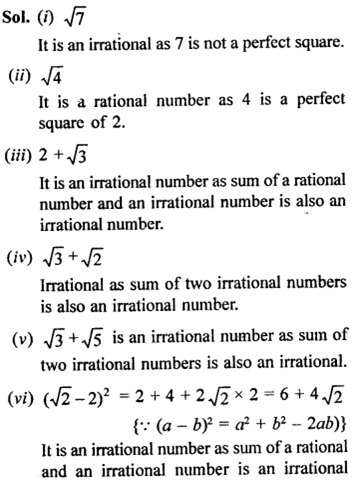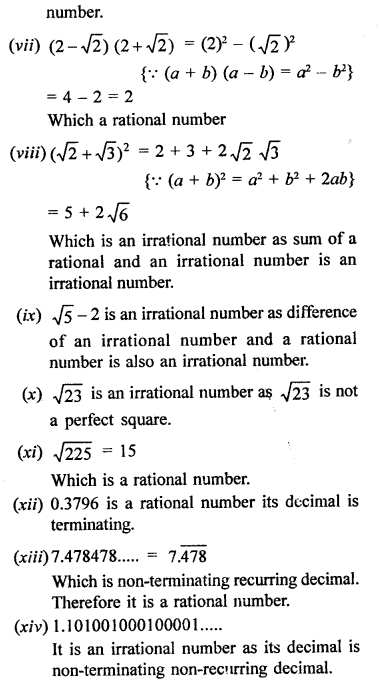Question 4 : Identify the following as rational or irrational numbers. Give the decimal representation of rational numbers: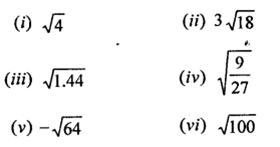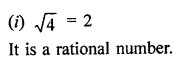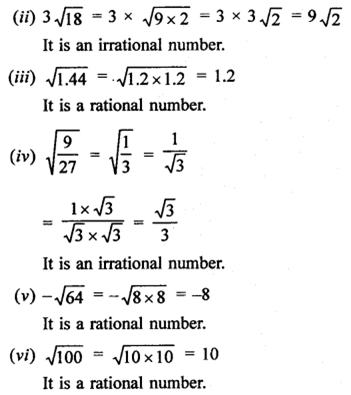Question 5 : In  the following equation, find which variables x, y, z etc. represent rational or irrational numbers: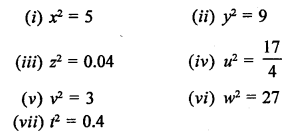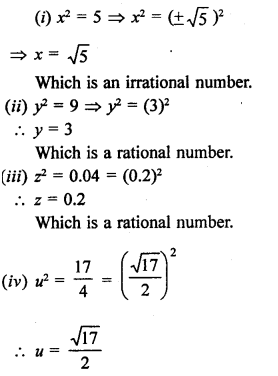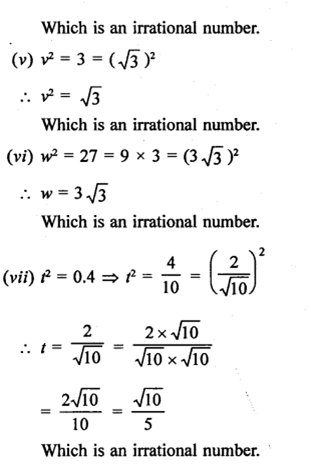Question 6 : Given two rational numbers lying between 0.232332333233332… and 0.212112111211112.

Answer 6 : Two rational numbers lying between 0.232332333233332… and 0.212112111211112… will be 0.232 and 0.212

Question 7 : Give two rational numbers lying between 0.515115111511115… and 0.5353353335…

Answer 7 : Two rational numbers lying between 0.515115111511115… and 0.535335333533335… will be 0.515, 0.535

Question 8 : Find one irrational numbers between 0.2101 and 0.2222… =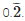Answer 8 : One irrational number lying between 0.2101 and 0.2222… =will be 2201.0010001…

Question 9 :
Find a rational number and also an irrational number lying between the numbers, 0.3030030003… and 0.3010010001…

Between two numbers 0.3030030003… and 0.3010010001…, a rational will be 0.301 and irrational number will be 0.3020020002…

Question 10 : Find three different irrational numbers between the rational numbers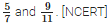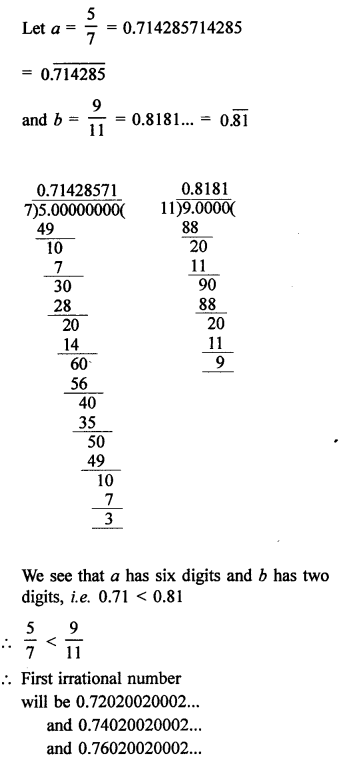Todays Deals### RD Chapter 1- Number Systems Ex-1.4 Contributorskrishan

Name:
Email:

# Latest News# 9000 interview questions in different categories